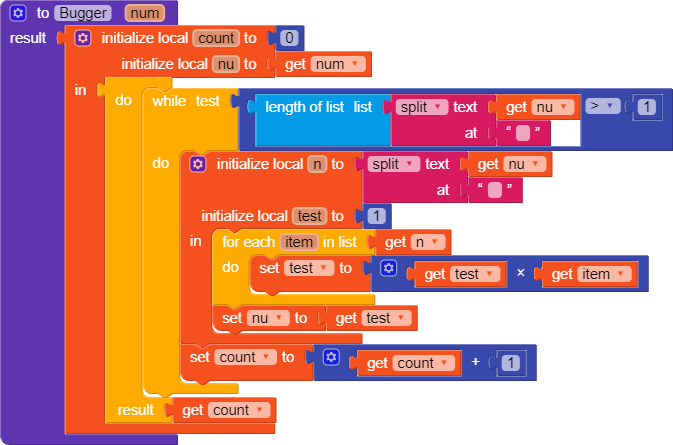# Daily Challenge #27

## Persistent Little Bugger

Create a procedure that takes a number and returns its multiplicative persistence , which is the number of times you must multiply the digits in `num` until you reach a single digit.

### Examples

``````bugger(39) ➞ 3
// Because 3 * 9 = 27, 2 * 7 = 14, 1 * 4 = 4 and 4 has only one digit.
bugger(999) ➞ 4
// Because 9 * 9 * 9 = 729, 7 * 2 * 9 = 126, 1 * 2 * 6 = 12, and finally 1 * 2 = 2.
bugger(4) ➞ 0
// Because 4 is already a one-digit number.``````
5 Likes

Here it is:untested
And here is JAVA code:

``````    public static int Bugger(int num){
int count = 0;
int nu = num;
while(Integer.toString(nu).length()>1){
String str = String.valueOf(nu);
String[] n = str.split("(?<=.)");
int test = 1;
for ( String i:n) {
test = Integer.parseInt(i)*test;
}
nu = test;
count ++;
}
return count;
}``````
2 Likes

Nice work. Can you show some screenshots of the results also?

4 Likes

Would’ve been easier to do had the WebViewer’s evaluate JS block been synchronous.

We could have converted the number (say 91234) into an expression (`9*1*2*3*4`), and then used JavaScript’s `eval()` function to evaluate the product.

3 Likes

This topic was automatically closed 30 days after the last reply. New replies are no longer allowed.